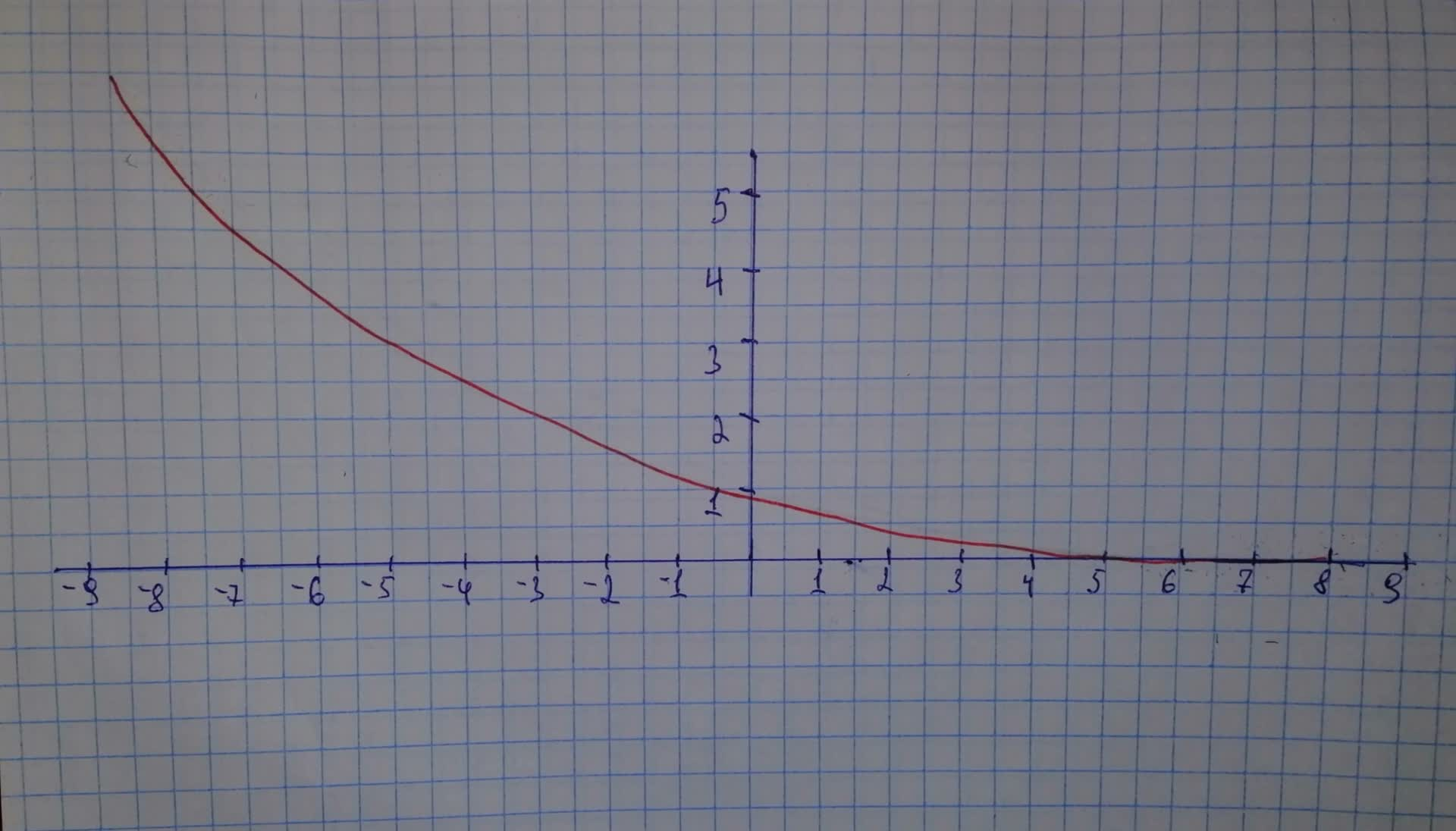# Determine whether the function represents exponential growth or exponential decay. Then graph the function. y=(0.8)^{x}Sinead Mcgee 2021-01-13 Answered
Determine whether the function represents exponential growth or exponential decay. Then graph the function.
$y={\left(0.8\right)}^{x}$
You can still ask an expert for help

## Want to know more about Exponential growth and decay?

• Questions are typically answered in as fast as 30 minutes

Solve your problem for the price of one coffee

• Math expert for every subject
• Pay only if we can solve itunessodopunsep

Step 1
Determine weather for the following function is represent exponential growth or exponential decay. Then graph the function:
$f\left(x\right)={\left(0.8\right)}^{x}$
There is a general rule for the exponential function depending on the base of exponent as follows:

For this function,
$\therefore$ This function is represent for exponential decay function.
Step 2